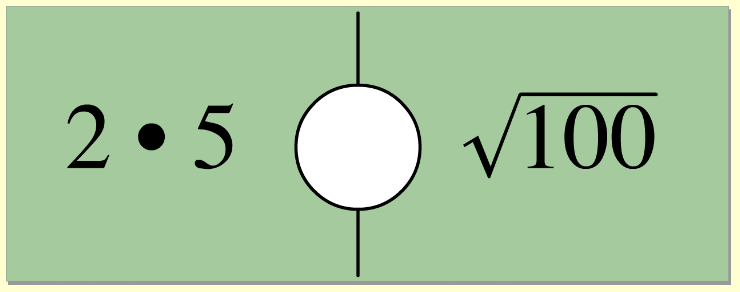Square Roots 1
Set 1 (10 Questions)
Students use exponents, powers, and roots, and use exponents in working with fractions
From Mr. Anker Tests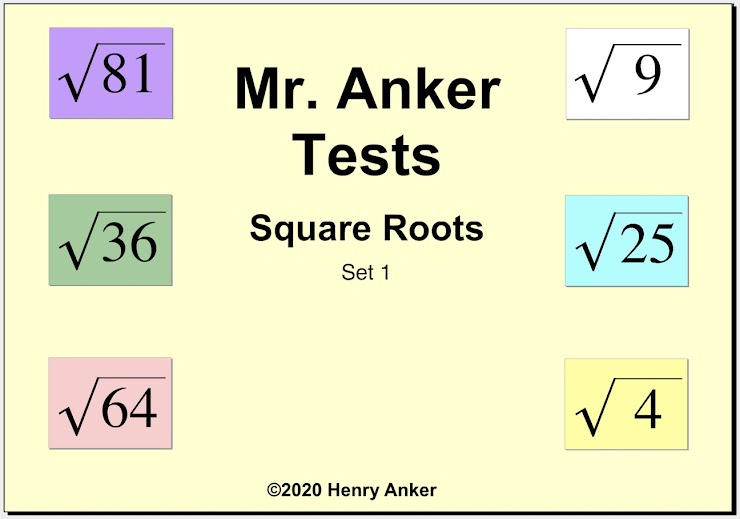Square Roots Help Slide 1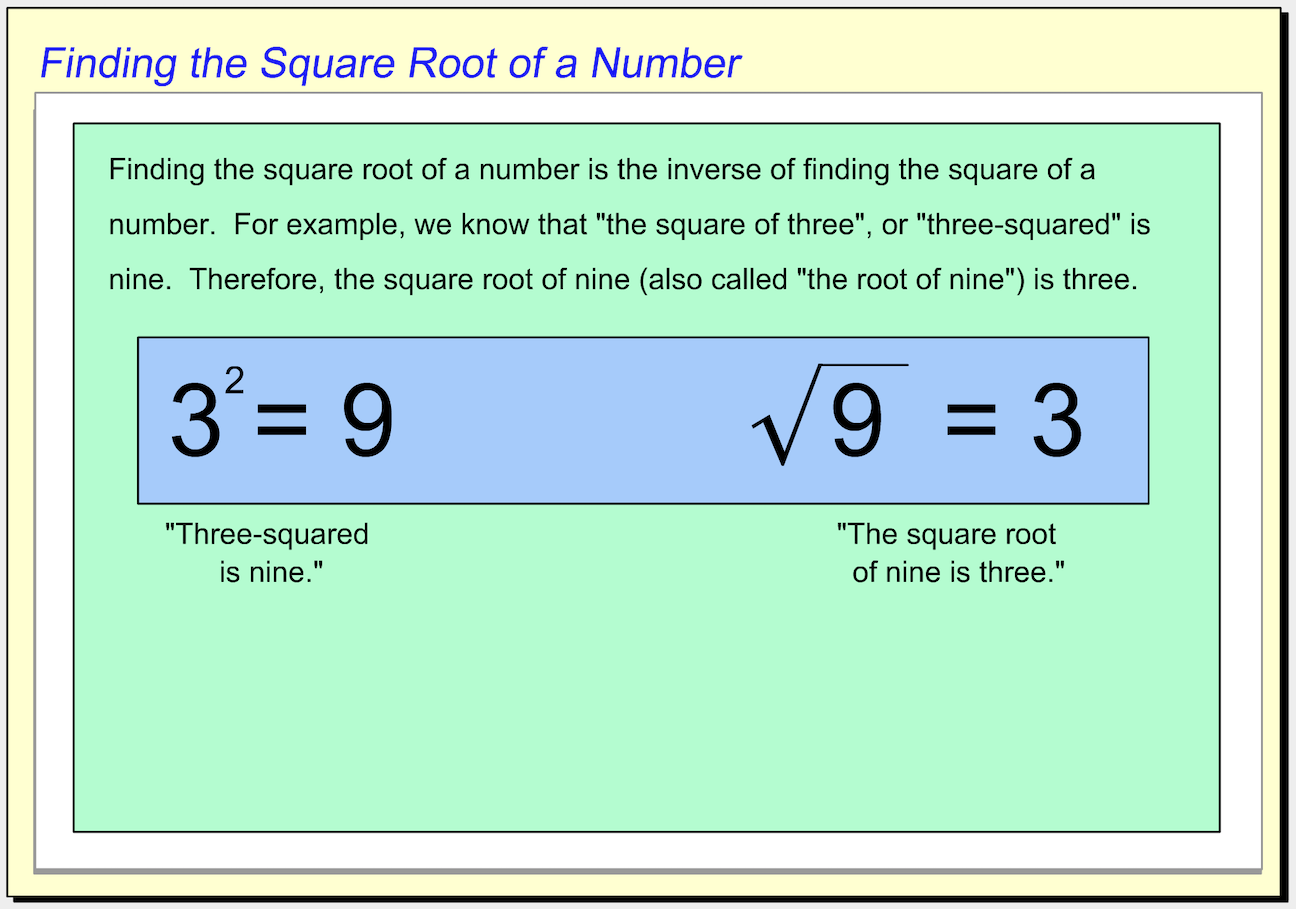Square Roots Help Slide 2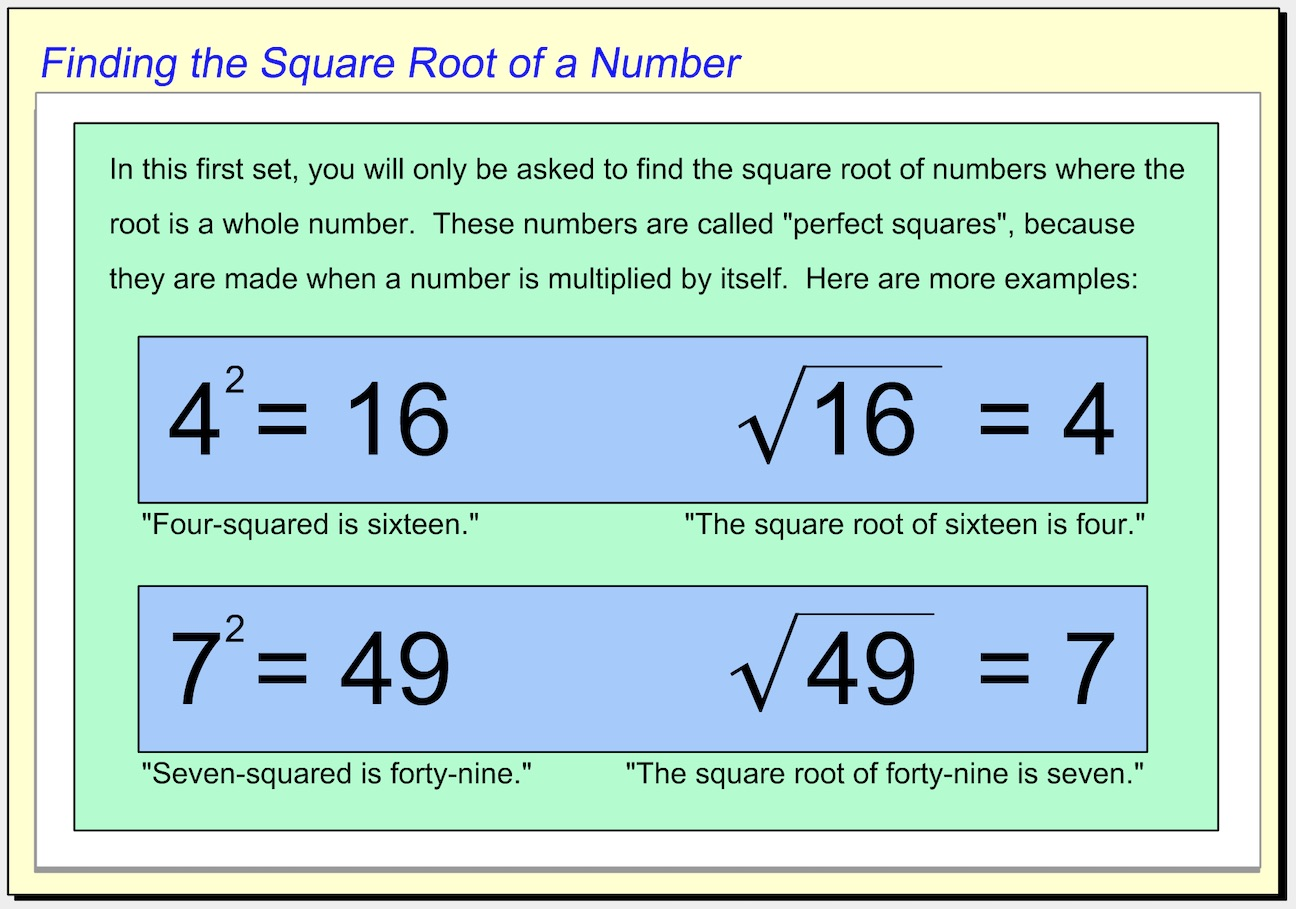Square Roots Help Slide 3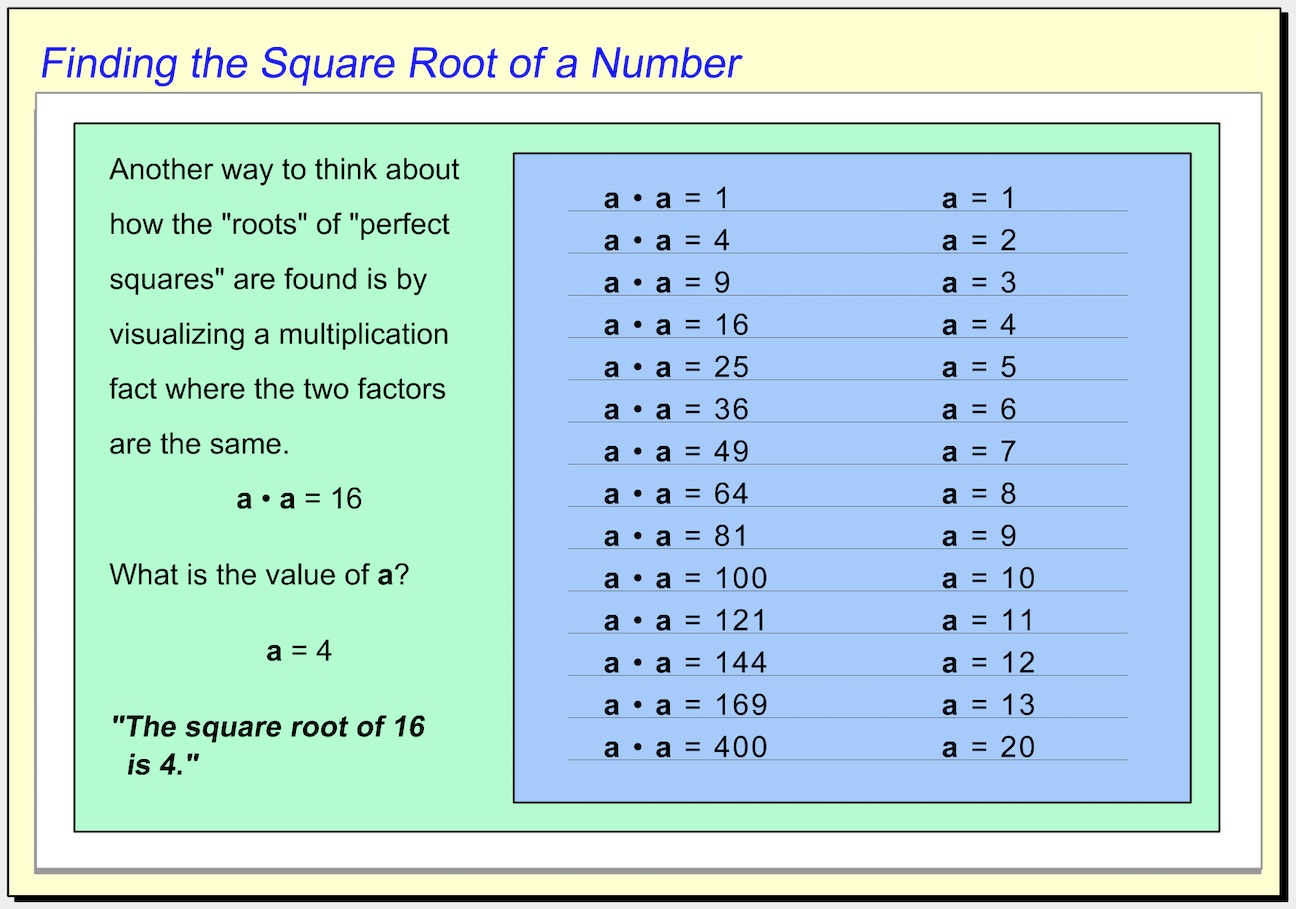Square Roots Help Slide 4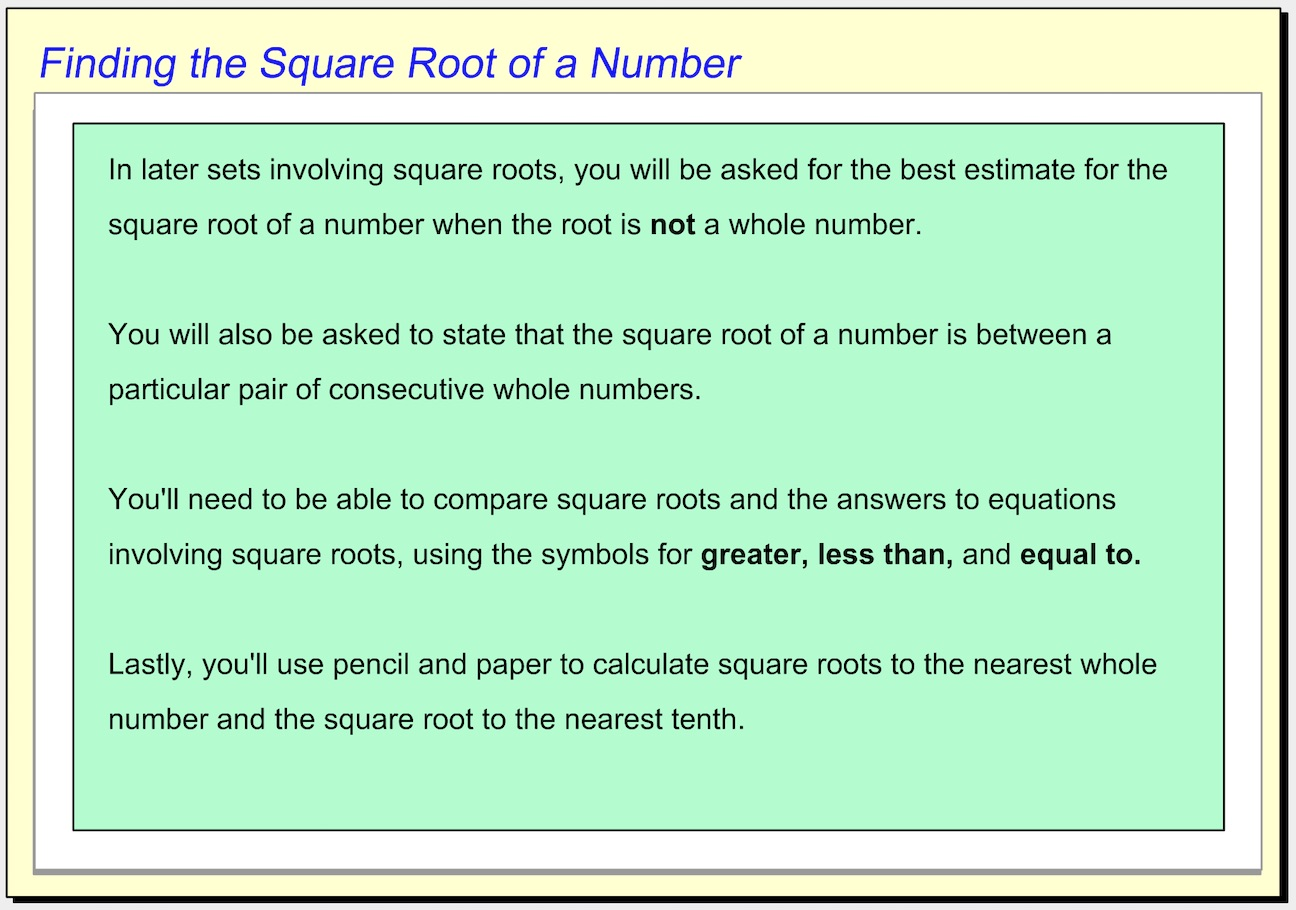1. Which choice shows the value of the square root displayed? *
1 point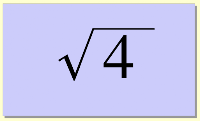2. Which choice shows the value of the square root displayed? *
1 point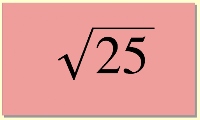3. Which choice shows the value of the square root displayed? *
1 point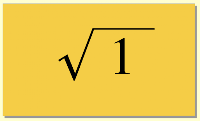4. Use the dropdown menu below to make this statement true: "Four is __________ the square root of 4." *
1 point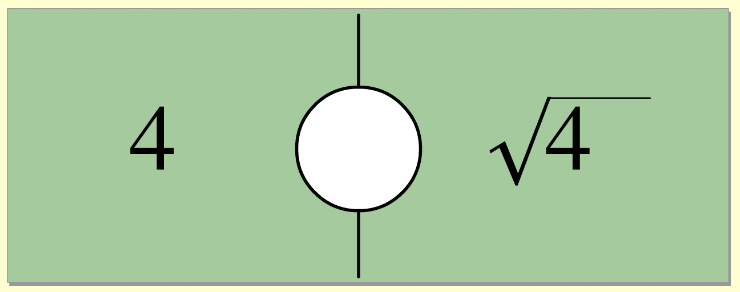5. Which choice shows the value of the square root displayed? *
1 point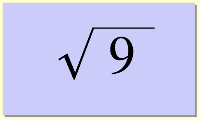6. Use the dropdown menu below to make this statement true: "The square root of 16 is __________ the square root of 25." *
1 point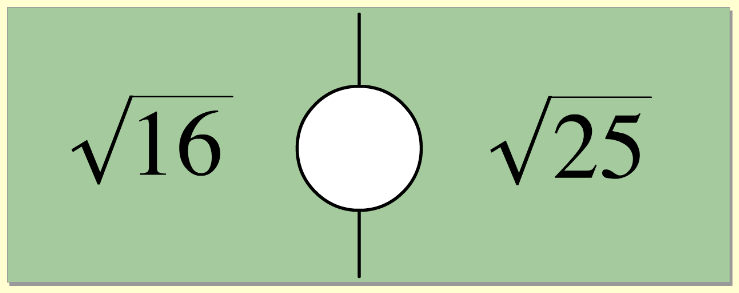7. Which choice shows the value of the square root displayed? *
1 point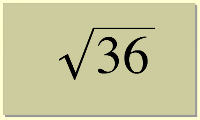8. Use the dropdown menu below to make this statement true: "Five is __________ the square root of 25." *
1 point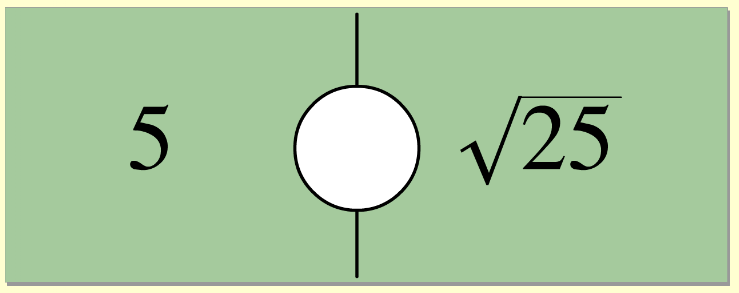9. On the line below, enter the equal value as a whole number. *
1 point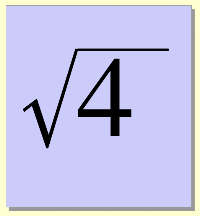10. Use the dropdown menu below to make this statement true: "The product of 2 times 5 is __________ the square root of 100." *
1 point